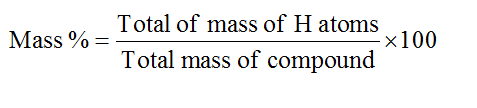Question
2 views

Determined the mass percentage of hydrogen in CH4 and C4H10?

check_circle

Step 1

Molar mass is defined as average mass of atoms present in the chemical formula. It is the sum of the atomic masses of all the atoms present in the chemical formula of any compound.

Step 2

The molar mass of CH4 and C4H10 is 16.04 g/mol and 58.12 g/mol respectively. The molar mass of H is 1.00784 g/mol.

Step 3

The mass percent of hydrogen in the given compound is calculated by the formula,...

### Want to see the full answer?

See Solution

#### Want to see this answer and more?

Solutions are written by subject experts who are available 24/7. Questions are typically answered within 1 hour.*

See Solution
*Response times may vary by subject and question.
Tagged in